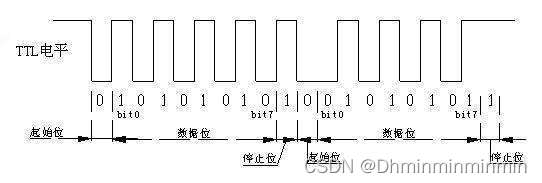# 【基于STM32的串口通信—-用软件模拟】

## 二：串口（串行接口）通信概念：

### 2、串口通信属于异步全双工模式，即可以在发送的同时进行接收，需要两根数据线（发送和接收），同时没有时钟线。## 三：串口通信TTL协议：## 四、keil软件里配置GPIO、写代码模拟协议（代码附下）

``````void GPIO_Init(void)
{
GPIO_InitTypeDef  GPIO_InitStructure;

RCC_AHB1PeriphClockCmd(RCC_AHB1Periph_GPIOD, ENABLE);//使能GPIOD时钟

//GPIOD9初始化设置
GPIO_InitStructure.GPIO_Pin = GPIO_Pin_9;//发送端TX对应IO口
GPIO_InitStructure.GPIO_Mode = GPIO_Mode_OUT;//普通输出模式
GPIO_InitStructure.GPIO_OType = GPIO_OType_PP;//推挽输出
GPIO_InitStructure.GPIO_Speed = GPIO_Speed_100MHz;//100MHz
GPIO_InitStructure.GPIO_PuPd = GPIO_PuPd_UP;//上拉
GPIO_Init(GPIOD, &GPIO_InitStructure);//初始化GPIO

GPIO_SetBits(GPIOD,GPIO_Pin_9);//GPIOD9设置高，灯灭

}

``````

``````void GPIO2_Init(void)
{
GPIO_InitTypeDef  GPIO_InitStructure;

RCC_AHB1PeriphClockCmd(RCC_AHB1Periph_GPIOD, ENABLE);//使能GPIOD时钟

//GPIOD12初始化设置
GPIO_InitStructure.GPIO_Pin = GPIO_Pin_12;//接收端RX对应IO口
GPIO_InitStructure.GPIO_Mode = GPIO_Mode_IN;//普通输入模式
GPIO_InitStructure.GPIO_Speed = GPIO_Speed_100MHz;//100MHz
GPIO_InitStructure.GPIO_PuPd = GPIO_PuPd_UP;//上拉
GPIO_Init(GPIOD, &GPIO_InitStructure);//初始化GPIO

GPIO_SetBits(GPIOD,GPIO_Pin_12);//GPIOD12设置高，灯灭

}

``````

``````#define TX_H GPIO_SetBits(GPIOD,GPIO_Pin_9)
#define TX_L GPIO_ResetBits(GPIOD,GPIO_Pin_9)

//发送一个字节的函数//
void Uart_tx_one_byte (u8 date)
{
int i;

TX_L;
delay_us(104);

for(i=0;i<8;i++)
{
if(date&(0x01<<i))
{
TX_H;
}
else
{
TX_L;
}
delay_us(104);
}

TX_H;
delay_us(104);
}

//发送多个字节//
void Uart_tx_bytes(u8 *pdata ,u8 len)
{
u8 i;
for(i=0;i<len;i++)
{
Uart_tx_one_byte(*(pdata+i));
}
}

``````

主函数里发送一个字节：

``````int main(void)
{
delay_init();//延时函数初始化//
GPIO_Init();
uart_init(9600);//波特率设置为9600//
while(1)
{
delay_ms(1000);

Uart_tx_one_byte(0x01);//发送0x01//
}
}

``````

``````u8 txbuf = {0x68,0x11,0x00,0x01,0x01,0x13,0x16};

int main(void)
{
delay_init();
GPIO_Init();
uart_init(9600);
while(1)
{
delay_ms(1000);

Uart_tx_bytes (txbuf,7);//将数组中的七个数发送出去//
}
}

``````

``````#define READ_LEVEL (GPIO_ReadInputDataBit(GPIOD,GPIO_Pin_12))

u8 ret;
u8 revdata,i;
u8 uart_rx(void)
{
if(ret == 0)
{
delay_us(10);//这里做个小延时，判断以免受到静电或者其他干扰，确保是起始位//
{
for(i=0;i<8;i++)
{
delay_us(104);
}
delay_us(104);
{
return 0;//接收成功返回0，其他情况返回0xff//
}
else
{
return 0xff;
}
}
else
{
return 0xff;
}
}
else
{
return 0xff;
}
}

``````

``````int main(void)
{
delay_init();
GPIO_Init();//TX端IO口初始化//
GPIO2_Init();//RX端IO口初始化//
uart_init(9600);
while(1)
{
delay_us(50);
if(uart_rx()==0)
{
Uart_tx_one_byte (0x10);//接收成功之后用TX端发送0x01，以供串口助手观察结果//
}
}
}

``````